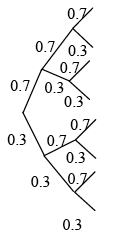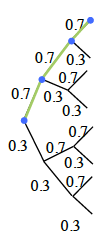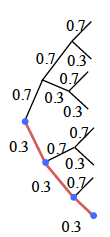### Home > PC > Chapter 7 > Lesson 7.3.3 > Problem7-122

7-122.

Álex Rodríguez gets a hit $30\%$ of the time.

1. Draw a tree diagram to show the possible outcomes of Rodríguez coming to bat three times.

Tree with 2 branches labeled 0.7 & 0.3, each splits into the same 2 branches, each of the 4 branches split into the same 2 branches again.

2. Find the probability Rodríguez gets $0$ hits.

$\left(0.7\right)^3$

Added to tree diagram, shading on 3 top branches each labeled 0.7.

3. Find the probability Rodríguez gets $3$ hits.

Added to tree diagram, shading on 3 bottom branches each labeled 0.3.

4. Find the probability Rodríguez gets $1$ hit.

3 possibilities. Find each and take their sum.

5. Find the probability Rodríguez gets $2$ hits.